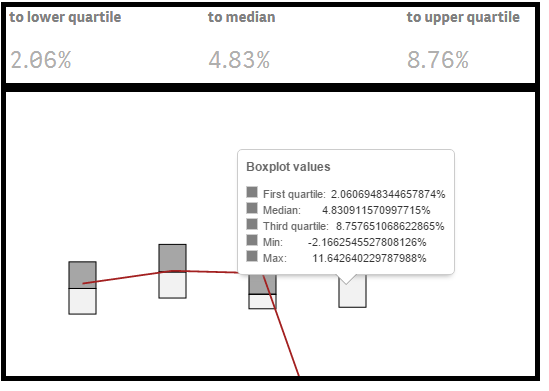# Qlik Sense App Development

Announcements
BARC’s The BI Survey 19 makes it official. BI users love Qlik. GET REPORT
Highlighted
New Contributor III

## How does AGGR function exactly work in case of missing values?

Hi Guys,

Perhaps you could help me here. I struggle to understand how exactly AGGR function works when I try to involve two aggr functions in one calculation.

Lets say I have the following formula:

aggr ( {< [Client TAG] = {'No'} >} sum( EBIT ) , ID , Year)

/

aggr ({ <[Client TAG] = {'No'} >} sum( Turnover ), ID , Year )

If there are no missing values this seems to work just fine and it gives a simple result (RESULT = outcome of the given formula)

aggr(EBIT)                aggr(TURNOVER)       RESULT

1                                 1                                  1

2                                 2                                  1

2                                 1                                  2

4                                 1                                  4

However, if there would be a missing value lets say in second row in Turnover, how this would look like in the AGGR temp tables calculation?

aggr(EBIT)                aggr(TURNOVER)       RESULT

1                                 1                                  1

2                                 1                                  2

2                                 1                                  2

4                                 -                                   -

OR

aggr(EBIT)                aggr(TURNOVER)       RESULT

1                                 1                                  1

2                                 -                                   -

2                                 1                                  2

4                                 1                                  4

Tags (4)
10 RepliesMVP

## Re: How does AGGR function exactly work in case of missing values?

If the denominator of a expression is null, what else would you expect the output to look like?

New Contributor III

## Re: How does AGGR function exactly work in case of missing values?

The fact that the outcome is NULL is obvious to me. I am rather asking about how will the further subtraction go.

I have a feeling that what happens is that it completely ignores the NULL values and if there is a NULL the temp table will have less rows and it can start subtracting wrong observations together

like in this example where the second ROW has missing turnover but in the end it is first three rows that get calculated due to the row three taking place at row 2.

aggr(EBIT)                aggr(TURNOVER)       RESULT

1                                 1                                  1

2                                 1                                  2

2                                 1                                  2

4                                 -                                   -MVP

## Re: How does AGGR function exactly work in case of missing values?

 The fact that the outcome is NULL is obvious to me. I am rather asking about how will the further subtraction go.

Further subtraction? Can you elaborate on this? I don't see any subtraction

New Contributor III

## Re: How does AGGR function exactly work in case of missing values?

I mean division not subtraction. Nevertheless the point is the same even if it was subtraction.MVP

## Re: How does AGGR function exactly work in case of missing values?

Subtraction can be handled using RangeSum()

RangeSum(

Aggr({<[Client TAG] = {'No'}>} Sum(EBIT), ID, Year)

-Aggr({<[Client TAG] = {'No'}>} Sum(Turnover), ID , Year)

)

Division can only happen if you divide by 1. May be you can do this

Aggr({<[Client TAG] = {'No'}>} Sum(EBIT), ID, Year)

/

Alt(Aggr({<[Client TAG] = {'No'}>} Sum(Turnover), ID, Year), 1)MVP

## Re: How does AGGR function exactly work in case of missing values?

There should be same number of rows for both nominator and denominator, because you are using same aggr() dimensions and set expression.

[edit: maybe this was a little too fast, it may depend on how your Turnover and EBIT field values are linked to ID and YEAR. ]

If in doubt, why not use

aggr ( {< [Client TAG] = {'No'} >} sum( EBIT ) /Sum(Turnover), ID , Year)

(and I would always consider using an outer aggregation function or explicitely stating Only() )

New Contributor III

## Re: How does AGGR function exactly work in case of missing values?

Thank you Stefan,

This is the kind of answer that I was hoping for. The ID and Year is linked to both EBIT and Turnover in exactly same way, they are just 2 different values in 2 different columns (simplified explanation).

The problem here however is that it still does not explain my problems that I am experiencing with the further use of this function.

I am actually using this further in my calculation to calculate upper, lower fractile and median out of those values. And what I am experiencing is that an extension for boxplots (I am forced to use an extension as the native QlikSense boxplot does not offer an option to add one more linear measure to the chart) simply shows different values for these once there are missing values than a simple KPI measure.

The difference in calculation is that for boxplot I simply calculate the Aggr and let the chart do the outer function in terms of calculating min,max,median,lower and upper Q. And for KPI I do manually calculate Fractile(aggr function, 0.25) etc..

Picture showing same results, no missing value in any of the observation,Picture showing different results, missing values have been in some of the observationsMVP

## Re: How does AGGR function exactly work in case of missing values?

Have you already tried to use a single Aggr() function as I suggested above?

New Contributor III

## Re: How does AGGR function exactly work in case of missing values?

‌HI Stefan,

Yes but the result it rather the same the only benefit that i see i brongs so far is that we create less temp tables thus have better performance.

Mismatch in the results remains the same though